# Pythagoras Tree

The first fractal I want to print is called the Pythagoras Tree. I love nature, so when I saw a mathematical expression of a tree I was intrigued. According to Wikipedia, it was created by Albert E. Bosman in 1942. Its name was derived from the Greek mathematician Pythagoras because it encloses so many right triangles, which are used to depict the Pythagorean theorem.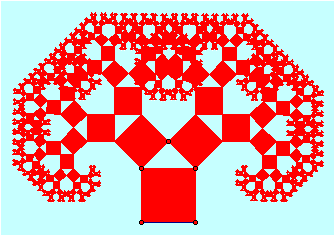The Pythagoras Tree is a 2D fractal that starts as a single square and is created from repeating squares. Larry Riddle explained the fractal very simply. Start with one square. Build a right isosceles triangle whose hypotenuse is the top edge of the square (making it look like a house in the image below). Then build two squares along each of the other two sides of this isosceles triangle.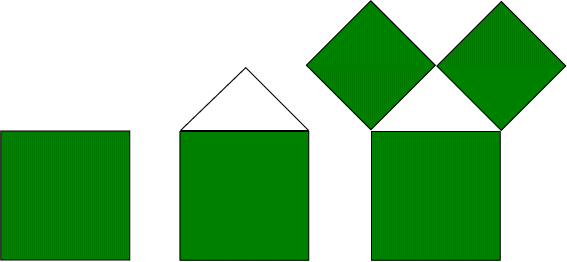Next, repeat this process onto each of the two new squares. As demonstrated in iteration 3, if you continue the process, a tree like construction will begin to form.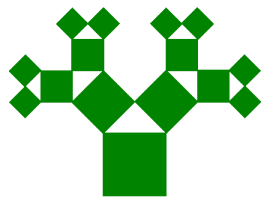I found a model on Thingiverse by blepfo, which I am slightly concerned about because he stated that he hasn’t actually printed it yet. But with that being said, I still think that it should print well because you can scale it down to whatever size you need and it doesn’t require a special printer.

## 3D Printing Results

The printing process was somewhat a challenge. My model had to be scaled down significantly otherwise it would have taken three hours to print. After scaling it down to 50mm, the printer started having difficulties because some of the squares were too small to physically print. As you can see in my picture, not all of the squares are present. I think if I had more time to print this fractal again, I would have more success.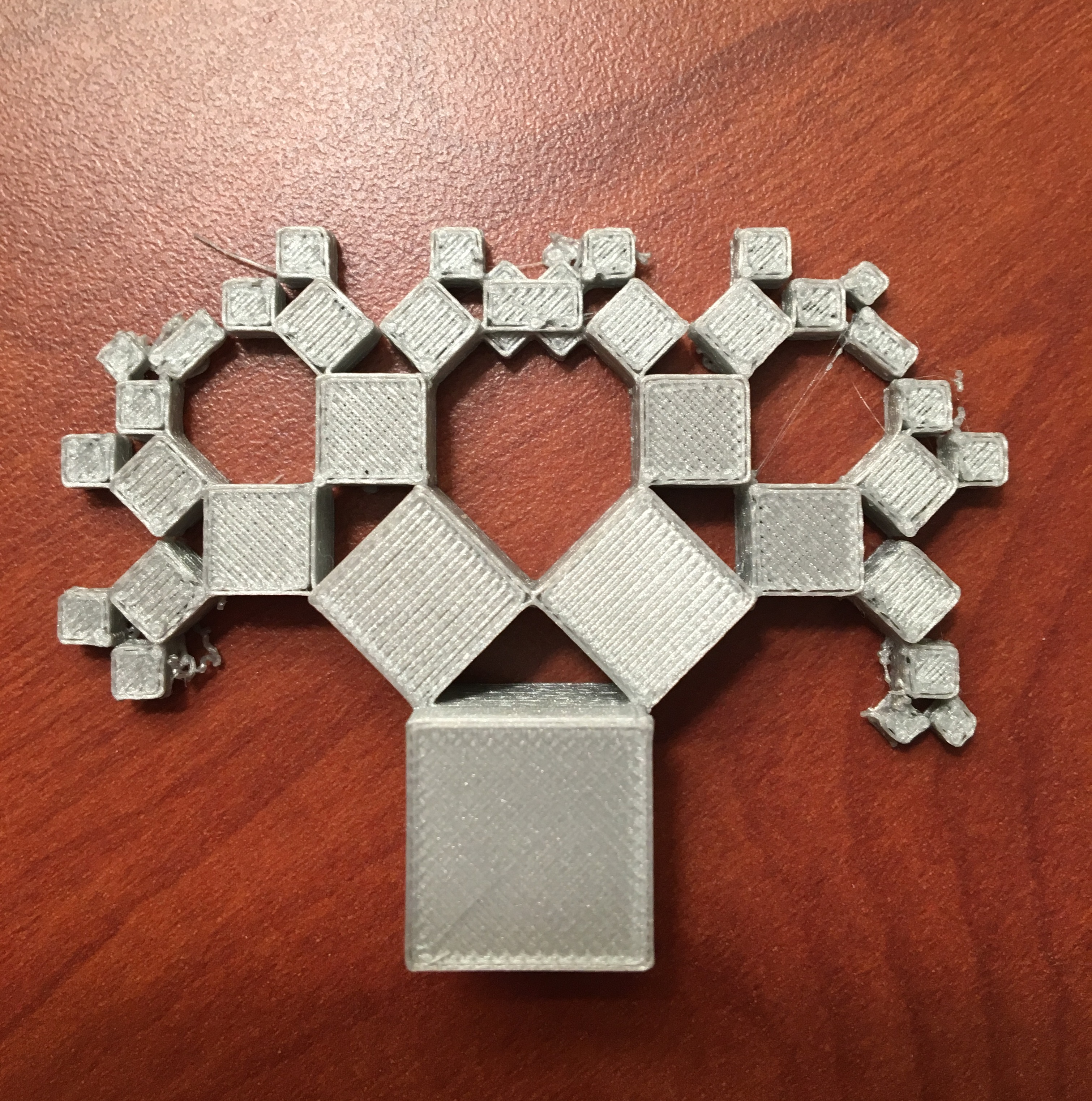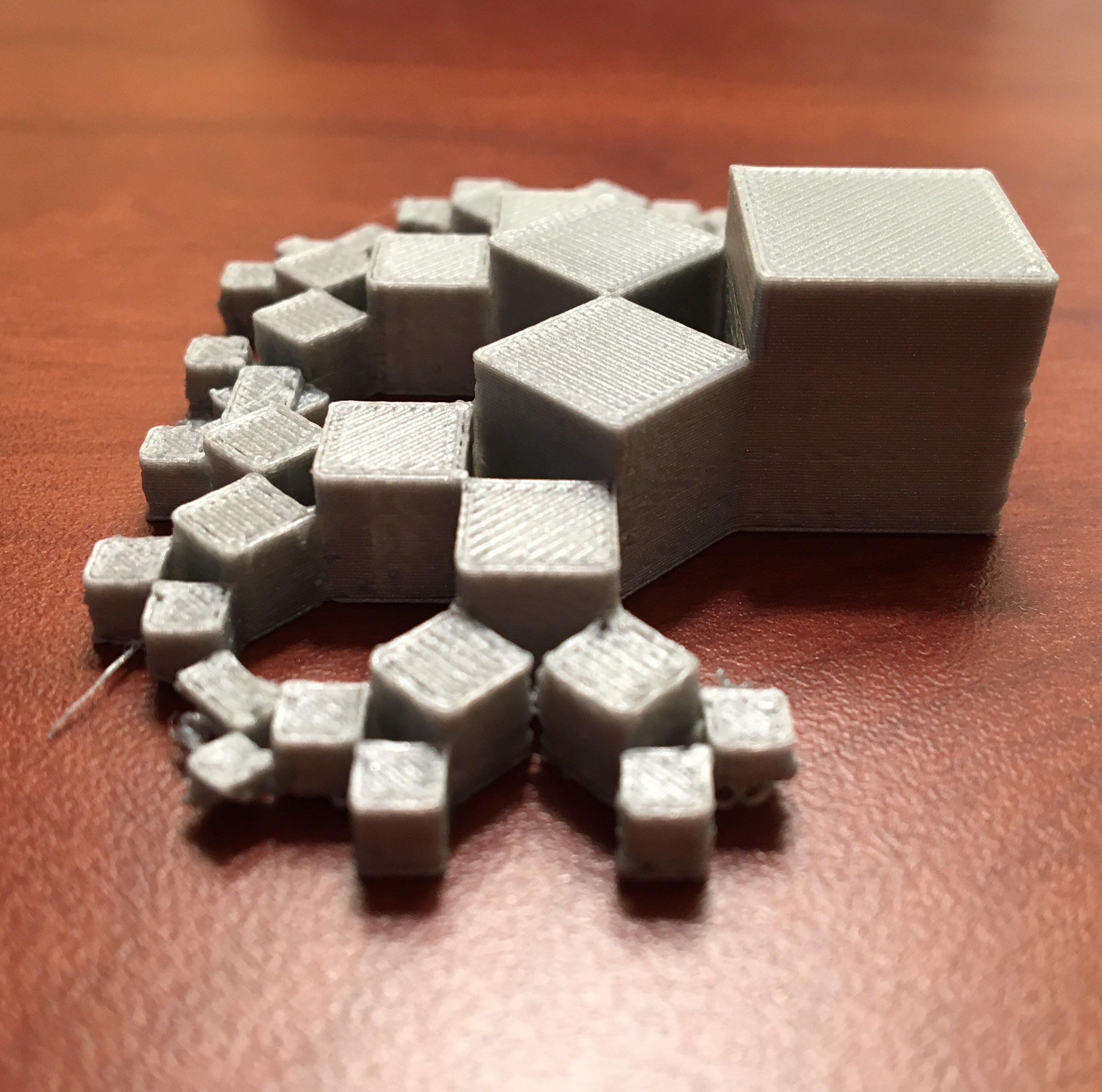#### 2 Comments on Pythagoras Tree

1. Don’t forget to post a Make and link it here

• What do you mean by this exactly?